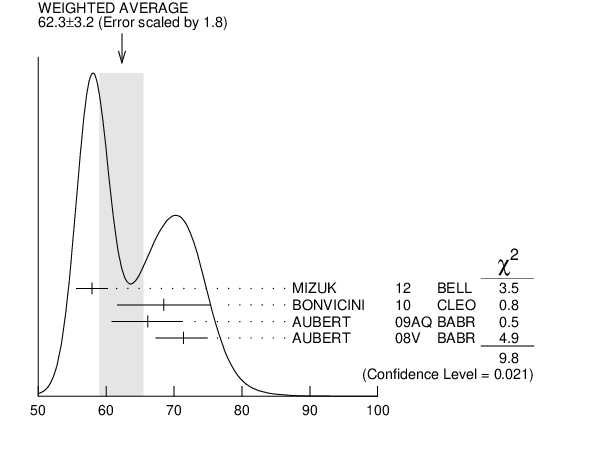# ${\boldsymbol m}_{{{\boldsymbol \Upsilon}{(1S)}}}–{\boldsymbol m}_{{{\boldsymbol \eta}_{{b}}}}$ INSPIRE search

VALUE (MeV) EVTS DOCUMENT ID TECN  COMMENT
$\bf{ 62.3 \pm3.2}$ OUR AVERAGE  Error includes scale factor of 1.8.
$57.9$ $\pm1.5$ $\pm1.8$ 34k 1
 2012
BELL ${{\mathit e}^{+}}$ ${{\mathit e}^{-}}$ $\rightarrow$ ${{\mathit \gamma}}{{\mathit \pi}^{+}}{{\mathit \pi}^{-}}$ + hadrons
$68.5$ $\pm6.6$ $\pm2.0$ $2.3$ $\pm0.5$k 2
 2010
CLEO ${{\mathit \Upsilon}{(3S)}}$ $\rightarrow$ ${{\mathit \gamma}}{{\mathit X}}$
$66.1$ ${}^{+4.8}_{-4.9}$ $\pm2.0$ $13$ $\pm5$k 2
 2009 AQ
BABR ${{\mathit \Upsilon}{(2S)}}$ $\rightarrow$ ${{\mathit \gamma}}{{\mathit X}}$
$71.4$ ${}^{+2.3}_{-3.1}$ $\pm2.7$ $19$ $\pm3$k 2
 2008 V
BABR ${{\mathit \Upsilon}{(3S)}}$ $\rightarrow$ ${{\mathit \gamma}}{{\mathit X}}$
• • • We do not use the following data for averages, fits, limits, etc. • • •
$67.1$ $\pm3.4$ $\pm2.3$ $10$ ${}^{+5}_{-4}$ 2, 3
 2012
${{\mathit \Upsilon}{(2S)}}$ $\rightarrow$ ${{\mathit \gamma}}$ hadrons
1  With floating width. Not independent of the corresponding mass measurement.
2  Assuming ${\Gamma}_{{\mathit \eta}_{{b}}{(1S)}}$ = 10 MeV. Not independent of the corresponding ${{\mathit \gamma}}$ energy or mass measurements.
3  Obtained by analyzing CLEO III data but not authored by the CLEO Collaboration.${\mathit m}_{{{\mathit \Upsilon}{(1S)}}}–{\mathit m}_{{{\mathit \eta}_{{b}}}}$ (MeV)
References:
 DOBBS 2012
PRL 109 082001 Observation of the ${{\mathit \eta}_{{b}}{(2S)}}$ Meson in ${{\mathit \Upsilon}{(2S)}}$ $\rightarrow$ ${{\mathit \gamma}}{{\mathit \eta}_{{b}}{(2S)}}$ , ${{\mathit \eta}_{{b}}{(2S)}}$ $\rightarrow$ Hadrons and Confirmation of the ${{\mathit \eta}_{{b}}{(1S)}}$ Meson
 MIZUK 2012
PRL 109 232002 Evidence for the ${{\mathit \eta}_{{b}}{(2S)}}$ and Observation of ${{\mathit h}_{{b}}{(1P)}}$ $\rightarrow$ ${{\mathit \eta}_{{b}}{(1S)}}{{\mathit \gamma}}$ and ${{\mathit h}_{{b}}{(2P)}}$ $\rightarrow$ ${{\mathit \eta}_{{b}}{(1S)}}{{\mathit \gamma}}$
 BONVICINI 2010
PR D81 031104 Measurement of the ${{\mathit \eta}_{{b}}{(1S)}}$ Mass and the Branching Fraction for ${{\mathit \Upsilon}{(3S)}}$ $\rightarrow$ ${{\mathit \gamma}}{{\mathit \eta}_{{b}}{(1S)}}$
 AUBERT 2009AQ
PRL 103 161801 Evidence for the ${{\mathit \eta}_{{b}}{(1S)}}$ Meson in Radiative ${{\mathit \Upsilon}{(2S)}}$ Decay
 AUBERT 2008V
PRL 101 071801 Observation of the Bottomonium Ground State in the Decay ${{\mathit \Upsilon}{(3S)}}$ $\rightarrow$ ${{\mathit \gamma}}{{\mathit \eta}_{{b}}}$